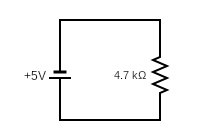## Current Flow

Calculate the current flowing through the below circuit diagram.Hint
Use Ohm’s law.
Hint 2
Ohm’s law:
$$V=IR$$$where $$V$$ is the voltage, $$I$$ is the current, and $$R$$ is the resistance Ohm's law: $$V=IR$$$
where $$V$$ is the voltage, $$I$$ is the current, and $$R$$ is the resistance

Solving for current $$(I)$$ :
$$I=\frac{V}{R}=\frac{5V}{4700\Omega}=0.001\:Amps$$\$
0.001 Amps